### Home > PC > Chapter 4 > Lesson 4.1.1 > Problem4-7

4-7.
1. Sketch each of the following angles onto a unit circle then find the coordinates of the angle. Homework Help ✎

1.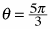2.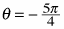3.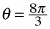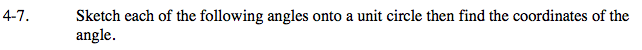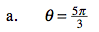The reference angle is π/3. What are the cosine and sine of π/3? When writing your final answer, remember that this angle is in the fourth quadrant.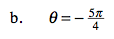1. Draw a unit circle.
2. Divide the top and bottom halves into quarters.
3. Put a mark on the unit circle at the 5 quarters going clockwise from (1,0).
4. Make a triangle with the x-axis.
5. Label the lengths of the sides.
6. Check for the correct signs.
7. The coordinates are (x, y).

What are the side lengths? Use the Pythogorean Theorem.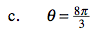$\frac{8\pi}{3}\text{ is the same angle as }\frac{8\pi}{3}-2\pi = \frac{2\pi}{3}$